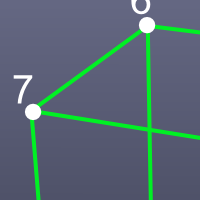# Manipulating Objects, Part 2

Last time I covered using the API to get component information about a polyShape. This time we’ll use the API to create a new polyShape cube.

Start by creating an instance of MFnMesh. This is the class we’ll use to create the cube. Specifically the create() function which has a few parameters we need to provide – the number of vertices, number of polygons, vertex positions, polygon vertex count, polygon connections and the new MObject for the resulting mesh.

In order to make the cube we must define the various components starting with the vertices. Here’s an image showing their build order. Make special note of this order as it’s import later on when connecting each polygon together.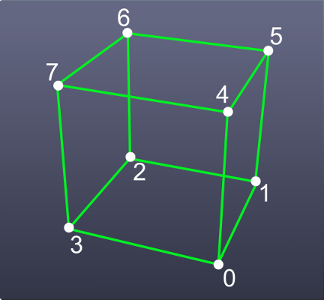We’re making a cube which means 8 vertices.

Next declare a variable containing an instance of MFloatPointArray. This will contain all the vertex positions of our cube and eventually will be passed into MFnMesh.create(). Each vertex position is defined using MFloatPoint and then appended to the MFloatPointArray variable. These positions are XYZ values in UI Units.

Now for the polygons. Here’s an image of the polygon build order.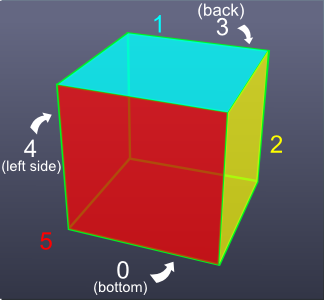Start by declaring a variable with the number of faces.

Then create an MIntArray() that contains the number of vertices per polygon of our cube. Since it’s a cube each poly will have four verts. In this example I use MScriptUtil to assign the ints to the MIntArray. Alternatively you could use MIntArray.setLength() (this would be the number of polygons) and MIntArray.set() to define that data.

Finally we need to specify how the polygons connect to each vertex. We know the number of polygons (6) and the number of vertices (8). Now we associate the vertices to each polygon. Visually it looks like this: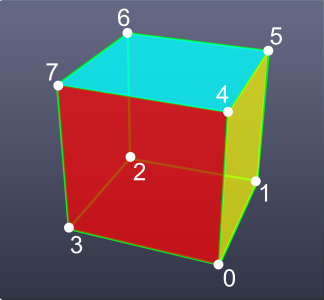Remember vertexArray? That variable we made earlier? Its indices are what we’ll be using. Create a variable vertPolyConnectsList which is a list. Each item in vertPolyConnectsList is an index from vertexArray. The order they are added is important. The first four entries (0,1,2,3) form the first polygon, the second four (4,5,6,7) the second polygon and so forth. You’ll notice in the code below that I put four vertex indices per line. This is a handy way to visualize each polygon (one per line).

Again we use MScriptUtil to set the polygon connection list into our MIntArray.

The last thing to do is create the mesh using MFnMesh.create(). The resulting MObject will be assigned into the variable objCube.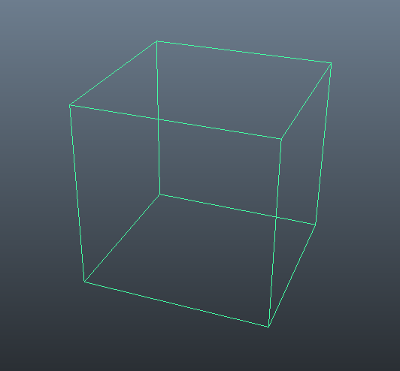Next time I’ll cover getting and setting UVs for a mesh.

Here is the complete code for this post.